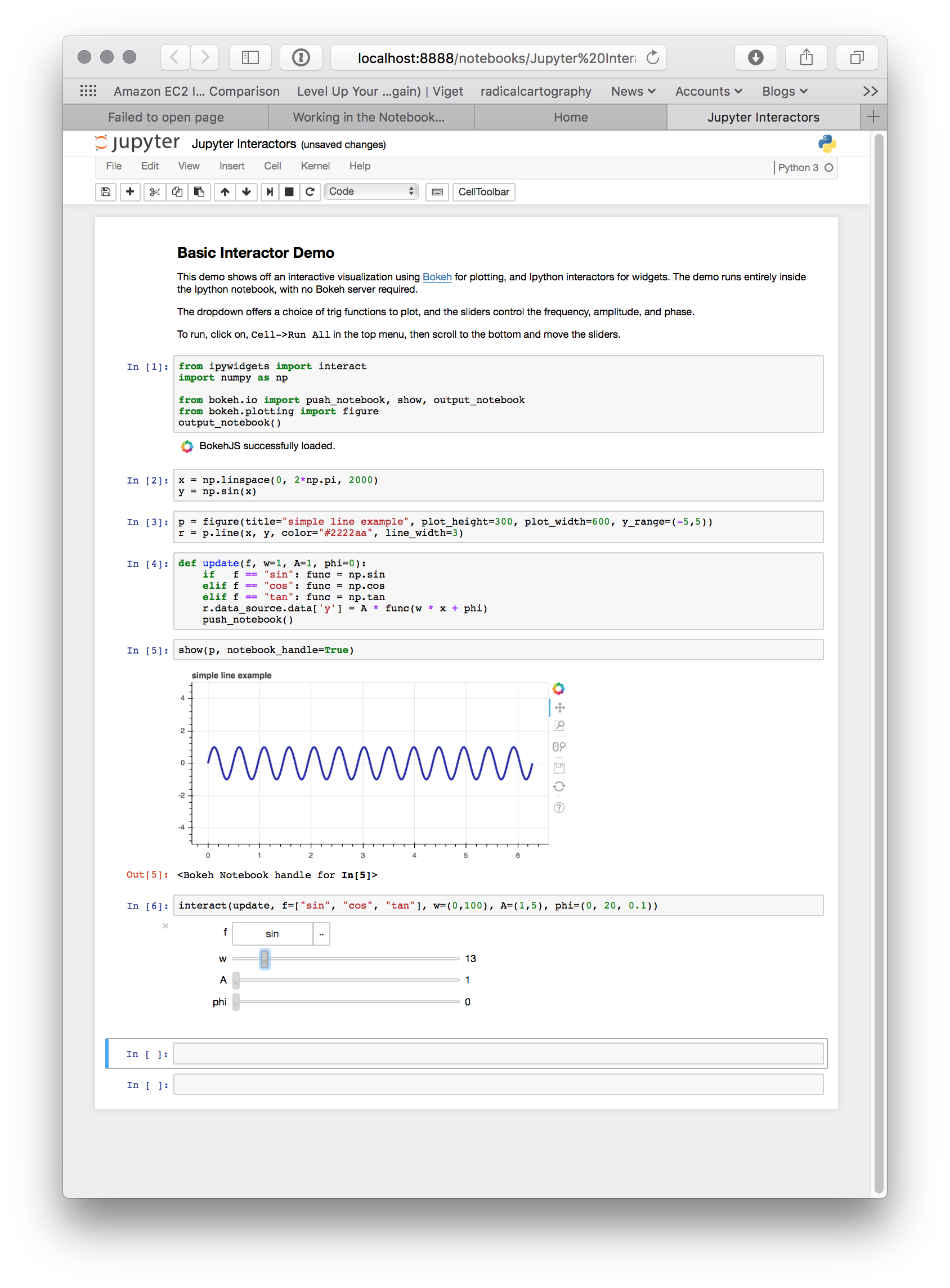# Working in the Notebook¶

## Inline Plots¶

To display Bokeh plots inline in an Jupyter notebook, use the `output_notebook()` function from bokeh.io instead of (or in addition to) the `output_file()` function we have seen previously. No other modifications are required. When `show()` is called, the plot will be displayed inline in the next notebook output cell. You can see a screenshot below: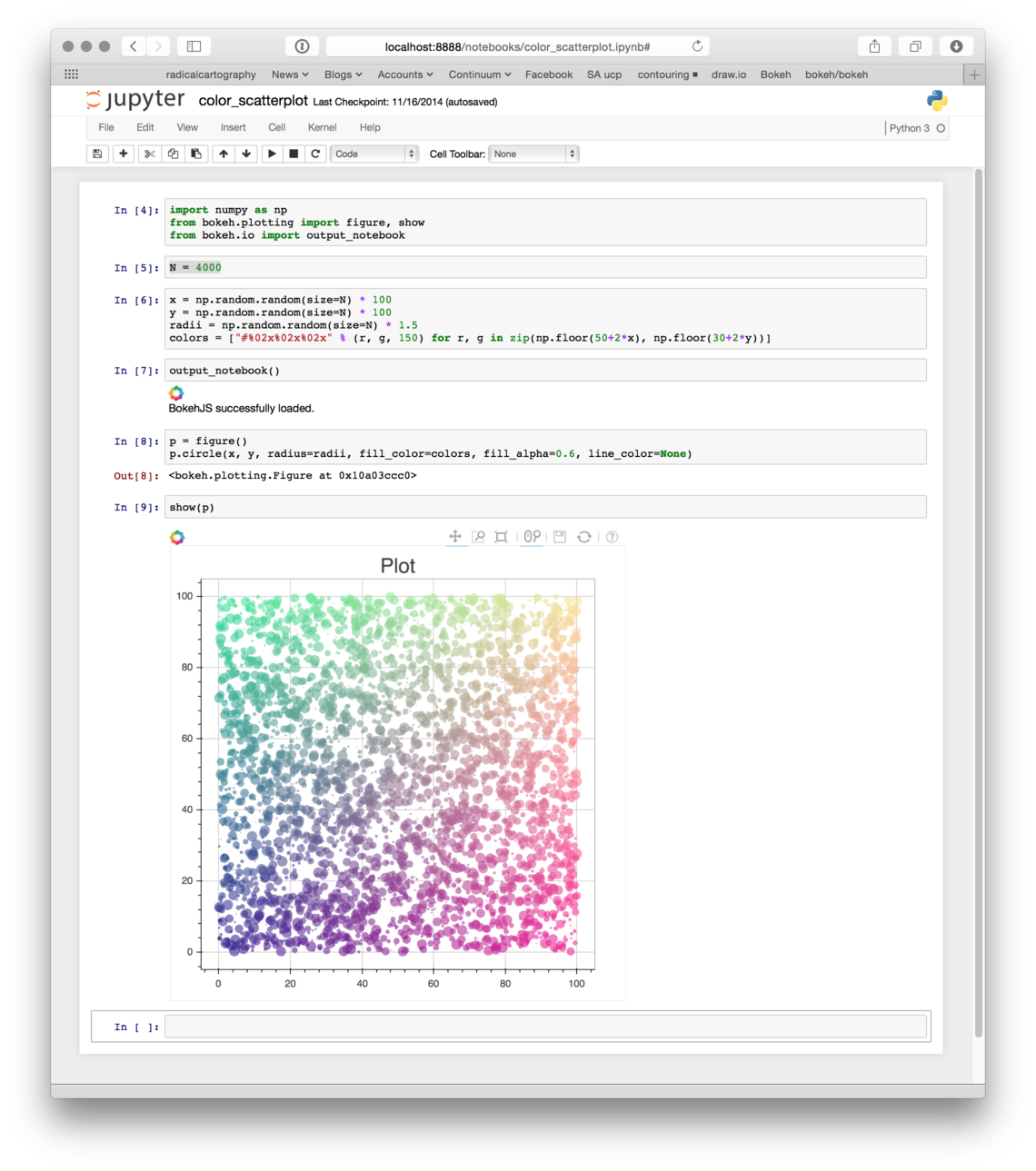Note

As a convenience, `output_notebook()` is also importable from the bokeh.charts and bokeh.plotting modules.

## Notebook Handles¶

It is possible to update a previously shown plot in-place. When the argument `notebook_handle=True` is passed to `show()` then a handle object is returned. This handle object can be used with the `push_notebook()` function to update the plot with any recent changes to plots properties, data source values, etc.

The following screenshots walk through the basic usage of notebook handles.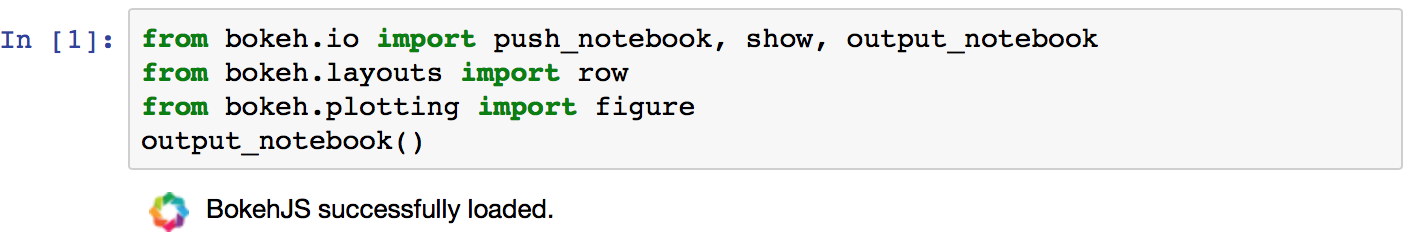• Next, create some plots, and make sure to pass `notebook_handle=True` to `show()`: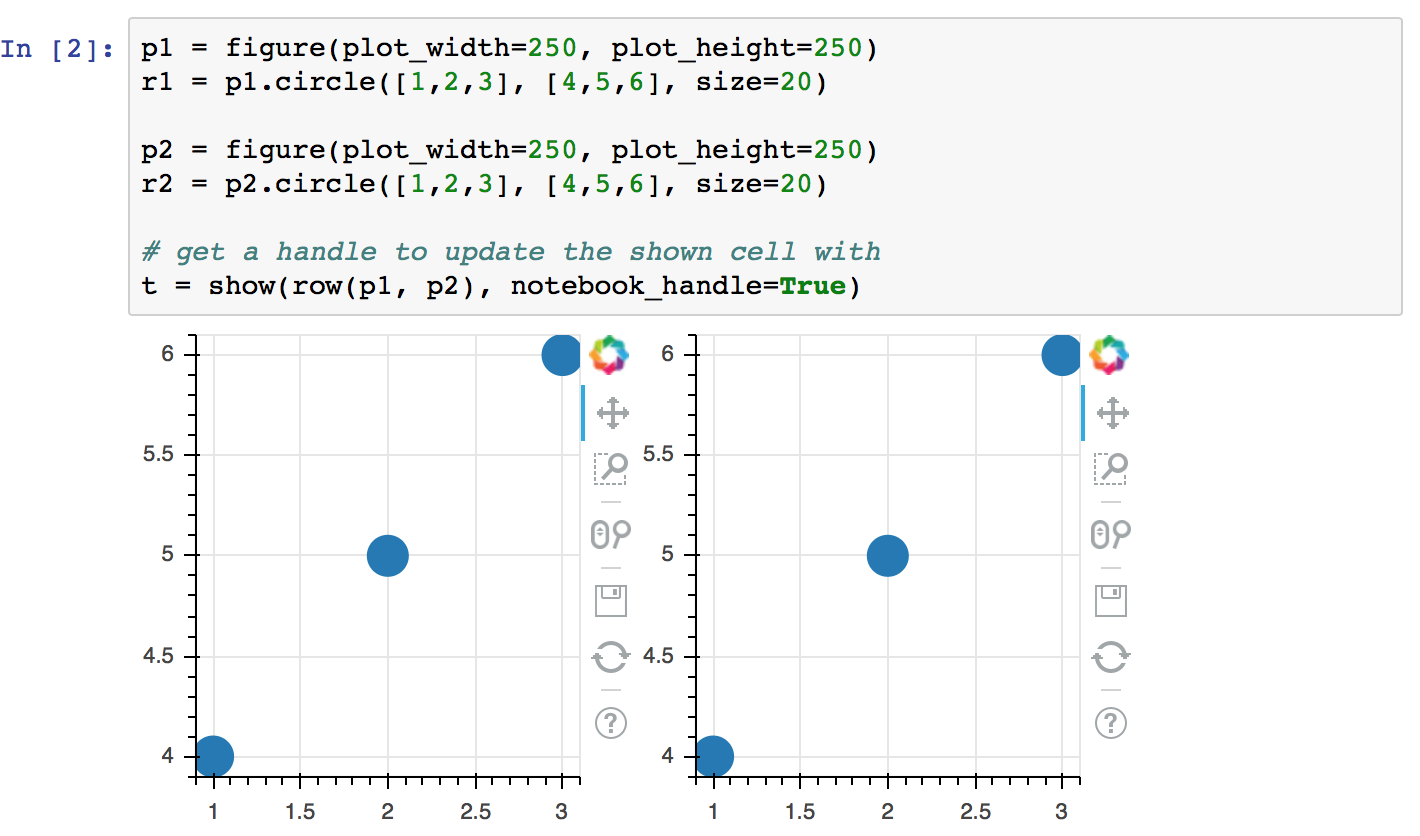• Looking at the handle, see that it is associated with the output cell for `In` that was just displayed: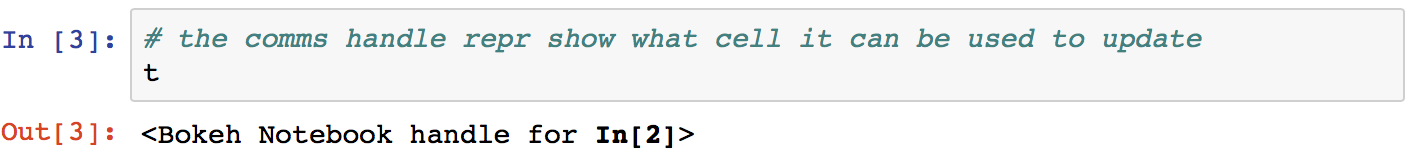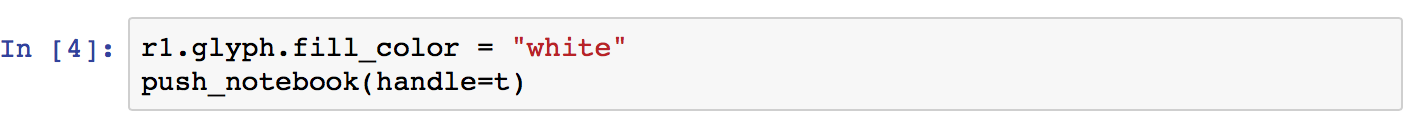• After doing so, note that the earlier output cell for `In` has changed (without being re-executed)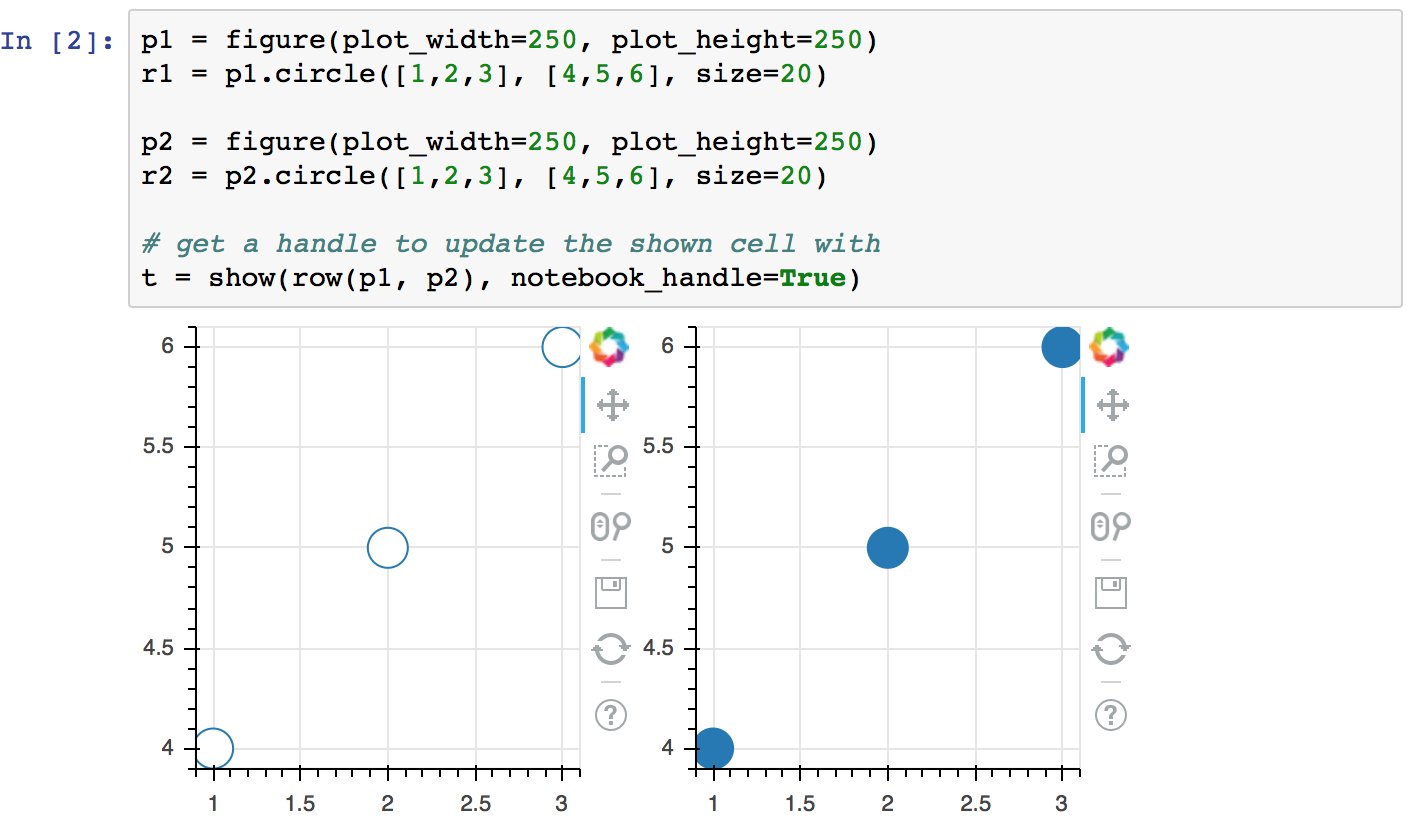More detailed demonstrations of using notebook handles can be found in the following example notebooks:

## Jupyter Interactors¶

It is possible to drive updates to Bokeh plots using Jupyter notebook widgets, known as interactors. The key doing this is the `push_notebook()` function described above. Typically it is called in the update callback for the interactors, to update the plot from widget values. A screenshot of the examples/howto/notebook_comms/Jupyter Interactors.ipynb example notebook is shown below: